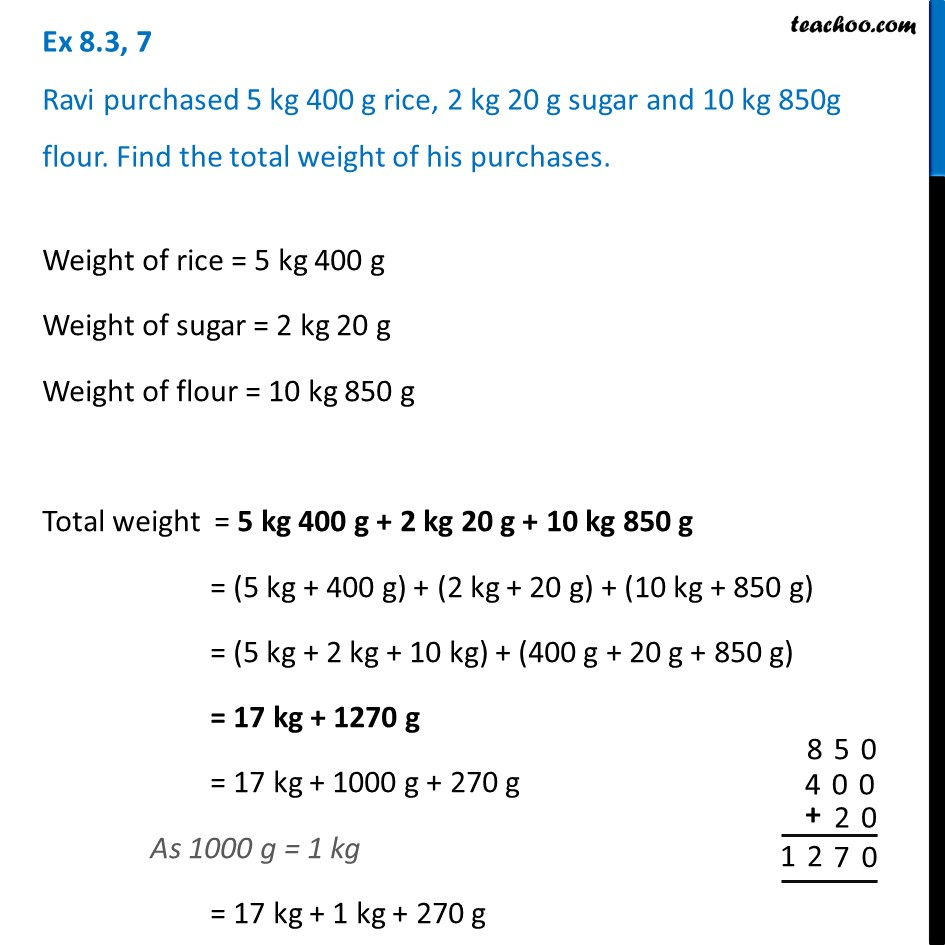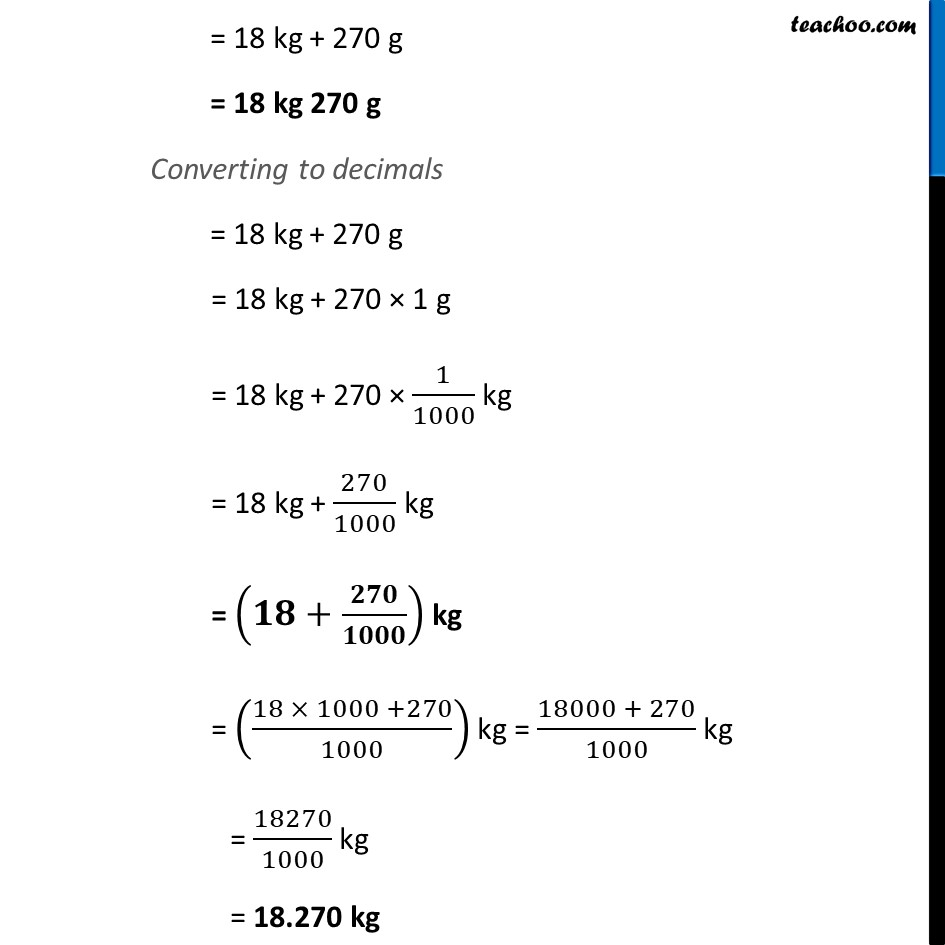Ex 8.3

Chapter 8 Class 6 Decimals
Serial order wiseLearn in your speed, with individual attention - Teachoo Maths 1-on-1 Class

### Transcript

Ex 8.3, 7 Ravi purchased 5 kg 400 g rice, 2 kg 20 g sugar and 10 kg 850g flour. Find the total weight of his purchases.Weight of rice = 5 kg 400 g Weight of sugar = 2 kg 20 g Weight of flour = 10 kg 850 g Total weight = 5 kg 400 g + 2 kg 20 g + 10 kg 850 g = (5 kg + 400 g) + (2 kg + 20 g) + (10 kg + 850 g) = (5 kg + 2 kg + 10 kg) + (400 g + 20 g + 850 g) = 17 kg + 1270 g = 17 kg + 1000 g + 270 g As 1000 g = 1 kg = 17 kg + 1 kg + 270 g